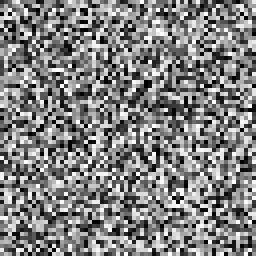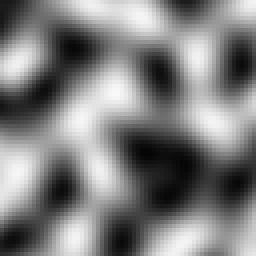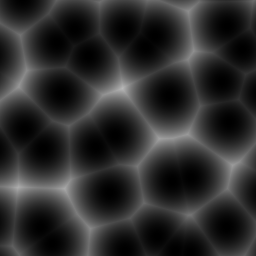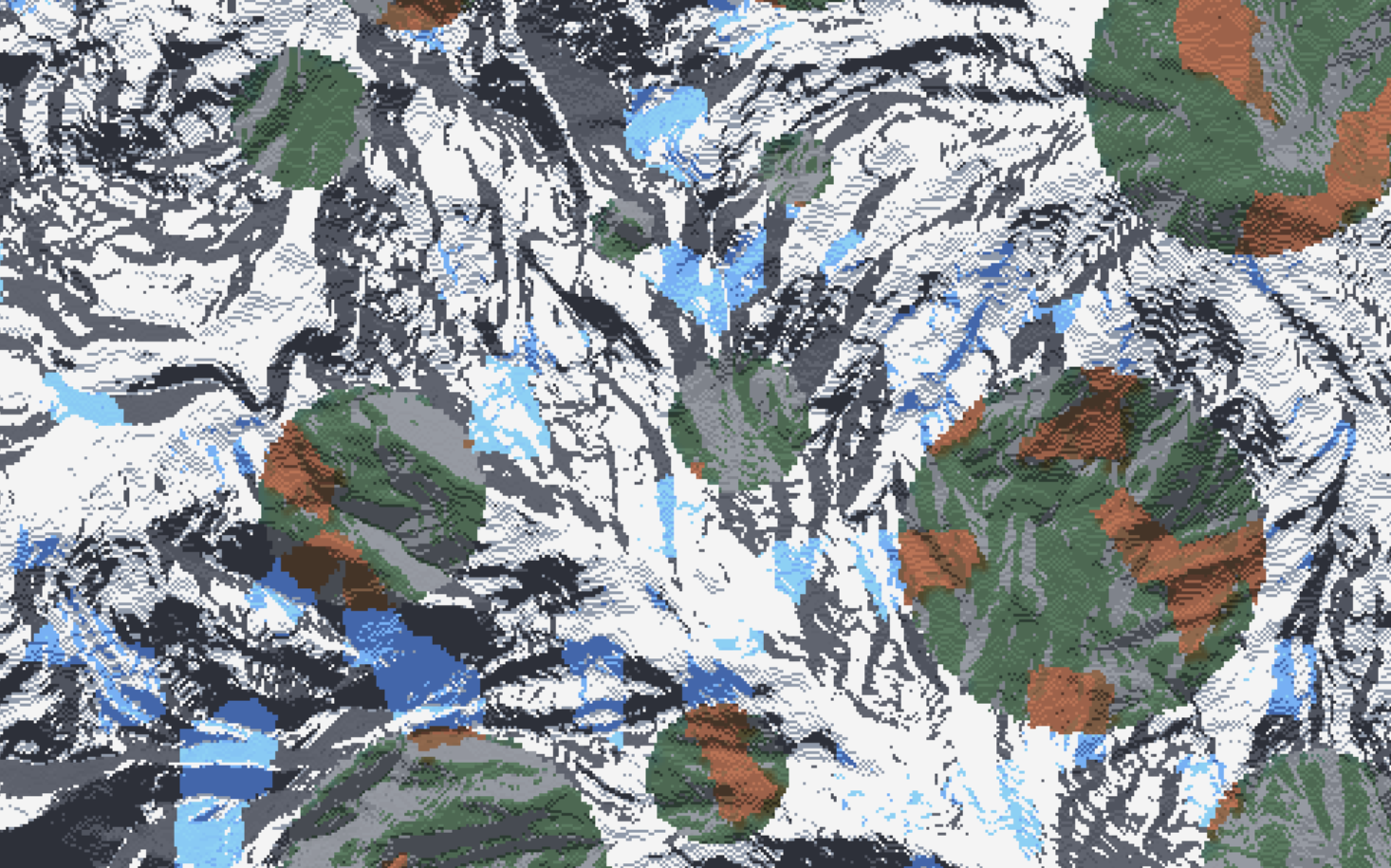# Sampler Configuration¶

A sampler is a config defined function that maps coordinates and the world seed to a set of output values.

A ‘sample’ is defined as a single calculation provided by a sampler, and the result of that calculation is regarded as the ‘output value’. A collective set of output values is regarded as ‘noise’.

The output values determine some kind of behaviour for each block or column. This behaviour is dependent on the context of the sampler definition.

## Shared Parameters¶

type `String` The ID of a sampler. Determines what kind of sampler is used, as well as what additional parameters become available to configure the sampler.

### DimensionApplicableSampler¶

The following is only required for `DimensionApplicableSampler`s

dimensions `Integer` How many dimensions the sampler operates. Must only be either `2`, or `3`.

• 2D samplers provide the same output value for blocks contained within the same world column (since the Y axis is not factored in 2D sampling).

• A 3D sampler defined in a parameter that expects a 2D sampler will result in the Y coordinate input defaulting to a constant value during sampling.

## Sampler Categories¶

Noise Samplers

Samplers that ‘produce noise’, i.e some pattern of values based on the input.

Random Noise Samplers

Samplers that use random values for every input, with no regard for producing any discernable structure. Outputs tend to look like tv-static.

Samplers where outputs produce structured noise. Inputs close in distance tend to produce similar output values.

Simplex Samplers

A group of samplers that use similar algorithms that produce outputs dubbed ‘simplex’ noise.

Mutator Samplers

Samplers that take other samplers as inputs. Mutator samplers typically act as ‘filters’ that provide some kind of modification to the output of other sampler(s).

Fractalizer Samplers

A category of mutator samplers that fractalize the input sampler.

Normalizer Samplers

A category of mutator samplers that redistribute the output values of a sampler.

Binary Arithmetic Samplers

Mutator samplers that combine the outputs of two samplers together using a math operator

Other Samplers

Other samplers that do not strictly fit into a single category.

## Sampler Types¶

### WHITE_NOISE¶

Produces White noise.frequency `Float` Controls the frequency of noise.

Default: `0.02`

salt `Integer` Determines the seed for the sampler.

Default: `0`

### POSITIVE_WHITE_NOISE¶

Identical to WHITE_NOISE, but redistributed to only produce positive values for convenience.

frequency `Float` Controls the frequency of noise.

Default: `0.02`

salt `Integer` Determines the seed for the sampler.

Default: `0`

### GAUSSIAN¶

Identical to WHITE_NOISE, but redistributed to follow a gaussian distribution.

frequency `Float` Controls the frequency of noise.

Default: `0.02`

salt `Integer` Determines the seed for the sampler.

Default: `0`

### PERLIN¶

Produces Perlin noise.

frequency `Float` Controls the frequency of noise.

Default: `0.02`

salt `Integer` Determines the seed for the sampler.

Default: `0`

Tip

It is recommended to use other simplex based samplers rather than PERLIN, as Perlin noise produces signficant directional artifacts, which may be undesired.

### SIMPLEX¶

Produces Simplex noise.

frequency `Float` Controls the frequency of noise.

Default: `0.02`

salt `Integer` Determines the seed for the sampler.

Default: `0`

### OPEN_SIMPLEX_2¶

Produces Simplex noise (using the algorithm from OpenSimplex2).frequency `Float` Controls the frequency of noise.

Default: `0.02`

salt `Integer` Determines the seed for the sampler.

Default: `0`

### OPEN_SIMPLEX_2S¶

Produces smoother Simplex noise (using the algorithm from OpenSimplex2).

frequency `Float` Controls the frequency of noise.

Default: `0.02`

salt `Integer` Determines the seed for the sampler.

Default: `0`

### VALUE¶

Produces Value noise using linear interpolation (bilinear for 2D, trilinear for 3D).

frequency `Float` Controls the frequency of noise.

Default: `0.02`

salt `Integer` Determines the seed for the sampler.

Default: `0`

### VALUE_CUBIC¶

Identical to VALUE except using cubic interpolation (bicubic for 2D, tricubic for 3D).

frequency `Float` Controls the frequency of noise.

Default: `0.02`

salt `Integer` Determines the seed for the sampler.

Default: `0`

### GABOR¶

Produces Gabor noise.

Warning

The GABOR sampler is significantly slower at producing noise compared to other noise samplers.

frequency `Float` Controls the frequency of noise.

Default: `0.02`

salt `Integer` Determines the seed for the sampler.

Default: `0`

rotation `Float`

Default: `0.25`

isotrophic `Boolean`

Default: `true`

deviation `Float`

Default: `1.0`

impulses `Float`

Default: `64.0`

frequency_0 `Float`

Default: `0.625`

### CELLULAR¶

Produces cellular / Worley noise.DIAGRAM• Black dots - The center of each cell.

• Red lines - A random direction and distance from the cell center, called ‘jitter’.

• Blue dots - The cell origin, determined by jitter from the cell center.

• Green dot - Coordinates being sampled.

• Purple line - Distance to the closest cell origin.

• Orange line - Distance to the second closest cell origin.

• Gold line - Distance to the third closest cell origin.

PARAMETERS

frequency `Float` Controls the frequency of noise.

Default: `0.02`

salt `Integer` Determines the seed for the sampler.

Default: `0`

distance `String` The method used for calculating the distance from the cell origin.

Default: `EuclideanSq`

Distance Types

• `Euclidean`

• `EuclideanSq`

• `Manhattan`

• `Hybrid`

return `String` The function the sampler will use to calculate the noise.

Default: `Distance`

Return Types

Definitions:

`s` - The coordinates being sampled.

`c` - The coordinates of the nearest cell origin.

`d1` - The distance from the nearest cell origin.

`d2` - The distance from the second nearest cell origin

`d3` - The distance from the third nearest cell origin

Types:

• `NoiseLookup` - Passes `c` into a sampler, and returns the output.

• `CellValue` - Returns a random value based on `c` (Equivalent to `NoiseLookup` with a WHITE_NOISE sampler).

• `Angle` - Returns the angle from `s` to `c`.

• `Distance` - Returns `d1`.

• `Distance2`- Returns `d2`.

• `Distance2Add` - Returns `(d1 + d2) / 2`.

• `Distance2Sub` - Returns `d2 - d1`.

• `Distance2Mul` - Returns `(d1 * d2) / 2`.

• `Distance2Div` - Returns `d1 / d2`.

• `Distance3` - Returns `d3`.

• `Distance3Add` - Returns `(d1 + d3) / 2`.

• `Distance3Sub` - Returns `d3 - d1`.

• `Distance3Mul` - Returns `d3 * d1`.

• `Distance3Div` - Returns `d1 / d3`.

jitter `Float` Determines how far cell origins can randomly spread out from the center of cells.

Default: `1`

A jitter of `0` places cell origins exactly in the center of each cell, resulting in a perfect grid. Values between `-1` and `1` are recommended, as values outside that range may produce artifacts.

lookup `Sampler` The lookup sampler used when the `distance` parameter is set to `NoiseLookup`

Default: OPEN_SIMPLEX_2 sampler

### IMAGE¶

Outputs the channel of an image that is tiled, redistributed from the channel range [0-255] to output range [-1, 1].

image `String` Path to the image relative to the config pack directory. (For Windows users: Use the `/` directory separator instead of `\`)

Example path: path/to/the/image.png

frequency `Float` Frequency of the image. Determines how the image gets scaled.

A frequency of `1.0` means 1 pixel = 1 block, a frequency of `2.0` means 2 pixels = 1 block.

Attention

Frequencies below `1.0` are not recommended, as pixels aren’t interpolated when upscaled; results may look pixelated depending on use.

`0.25` frequency = `0.25 pixels = 1 block` or `1 pixel = 4 blocks` (as demonstrated above using a block grid).

channel `String` Which channel of the image to output.

Valid channels:

• `GRAYSCALE`

• `ALPHA`

• `RED`

• `GREEN`

• `BLUE`

Channel Examples

Original ImageGrayscaleAlpha Channel*Red ChannelGreen ChannelBlue Channel*The alpha channel is all white because there is no transparency in the original image.

Example Image Samplers

World generated using the mountain heightmap to shape the terrain, and the circles to determine biome temperature:Terrain Sampler (Using LINEAR_HEIGHTMAP to work as a terrain sampler)

```type: LINEAR_HEIGHTMAP
base: 128
scale: 64
sampler:
type: IMAGE
image: mountain_heightmap.png
channel: GRAYSCALE
frequency: 1
```

Temperature Sampler

```type: IMAGE
image: grayscale_circles.png
channel: GRAYSCALE
frequency: 1
```

### CONSTANT¶

Outputs a constant value, regardless of the inputs. Typically used in cases where you don’t want the sampler to do anything.

value `Float` The value to be outputted.

Default: `0.0`

### DOMAIN_WARP¶

Warps a sampler by another sampler. See Domain Warping for more information.

warp `Sampler` The sampler that determines warping.

sampler `Sampler` The sampler to be warped.

amplitude `Float` How much warping to apply.

Default: `1.0`

### KERNEL¶

kernel `List` of `List` of `Float`

sampler `Sampler`

factor `Float`

Default: `1.0`

frequency `Float`

Default: `1.0`

### LINEAR_HEIGHTMAP¶

Treats a 2D sampler as a heightmap, converting it to a 3D SDF for use as a terrain sampler.

base `Float` The base y level of the terrain.

sampler `Sampler` The sampler to be used as a heightmap.

Default: CONSTANT sampler

scale `Float` Scales the height of the heightmap.

Default: `1.0`

### FBM¶

sampler `Sampler`

octaves `Integer`

Default: `3`

gain `Float`

Default: `0.5`

lacunarity `Float`

Default: `2.0`

weighted-strength `Float`

Default: `0.0`

### PING_PONG¶

sampler `Sampler`

octaves `Integer`

Default: `3`

gain `Float`

Default: `0.5`

lacunarity `Float`

Default: `2.0`

weighted-strength `Float`

Default: `0.0`

ping-pong `Float`

Default: `2.0`

### RIDGED¶

sampler `Sampler`

octaves `Integer`

Default: `3`

gain `Float`

Default: `0.5`

lacunarity `Float`

Default: `2.0`

weighted-strength `Float`

Default: `0.0`

### LINEAR¶

Redistributes the range `[min, max]` to `[-1, 1]`, typically for use with weighted pools.

sampler `Sampler`

max `Float`

min `Float`

### CLAMP¶

Outputs `max` when the sampler outputs a value greater than `max`, and returns `min` when the sampler outputs a value less than `min`, used to constrain sampler outputs to a certain range.

sampler `Sampler`

max `Float`

min `Float`

### NORMAL¶

Redistributes normally distributed outputs to be evenly distributed.

sampler `Sampler`

mean `Float`

standard-deviation `Float`

groups `Integer`

Default: `16384`

### PROBABILITY¶

Redistributes the range `[-1, 1]` to `[0, 1]`, typically used in cases where it’s easier to work with values from `0` to `1`, e.g defining a threshold as a percentage of a noise sampler.

sampler `Sampler`

### SCALE¶

Evaluates `sampler() * amplitude`.

sampler `Sampler`

amplitude `Float`

### POSTERIZATION¶

Applies a step function to the sampler, where `steps` determines how many steps will be within the range `[-1, 1]`.

sampler `Sampler`

steps `Integer`

Evaluates `left() + right()`.

left `Sampler`

right `Sampler`

### SUB¶

Evaluates `left() - right()`.

left `Sampler`

right `Sampler`

### MUL¶

Evaluates `left() * right()`.

left `Sampler`

right `Sampler`

### DIV¶

Evaluates `left() / right()`.

left `Sampler`

right `Sampler`

### MAX¶

Evaluates `max(left(), right())`.

left `Sampler`

right `Sampler`

### MIN¶

Evaluates `min(left(), right())`.

left `Sampler`

right `Sampler`

### EXPRESSION¶

Evaluates an arbitrary user defined expression as the sampler output. Expression samplers additionally allow the use of user defined functions, including other samplers, as well as constants defined within the sampler.

expression `String` The expression to be evaluated for each sample. Variables `x`, `y` (3D only), and `z` act as the sampler’s coordinate inputs.

Expression example

```expression: (x * 3) / z
```

variables `Map` < `String` : `Float` > Defines a mapping of variable names to values for use in the scope of the expression. This is most useful for providing named constants that can easily be modified if needed.

Default: Empty map

Example defining variables

```variables:
a: 1
b: 2
```

samplers `Map` < `String` : `DimensionApplicableSampler` > Defines a mapping of function names to samplers.

Each sampler may be utilized within the expression like so: `<name>(<x>, <z>)` (for 2D) or `<name>(<x>, <y>, <z>)` (for 3D), where <name> is the declared function name, and where the respective axis coordinates are the coordinate inputs passed to the sampler.

Default: Empty map

Note

Samplers defined within an EXPRESSION sampler must be `DimensionApplicableSampler`s, see the DimensionApplicableSampler section for details.

Example defining samplers

```samplers:
whiteNoise:
dimensions: 2
type: WHITE_NOISE
```

functions `Map` < `String` : `MathFunction` > Defines a mapping of function names to user-defined math functions. Functions may be called within the expression like so `<name>(<arg 1>, <arg 2>, ...)`, where <name> is the declared function name, and where each input coorresponds to the argument list defined by the function.

Default: Empty map

Example defining functions

```functions:
arguments:
- a
- b
- c
expression: (a + b) / c
```
Example Expression Samplers
```type: EXPRESSION

expression: 1 + 1
```

The sampler above outputs the result of `1` plus `1`, therefore the sampler will always output `2`.

Using variables
```type: EXPRESSION

variables:
a: 1
b: 2

expression: a - b
```

The sampler above outputs the result of `a` minus `b`, which is evaluated as `1` minus `2`, therefore the sampler will always output `-1`.

Using functions
```type: EXPRESSION

functions:
arguments:
- a
- b
- c
expression: (a + b) / c

expression: addThenDivide(3, 2, 10)
```

The sampler above outputs the results of passing `3`, `2`, and `10` into the function `addThenDivide()`. This function evaluation would be `(3 + 2) / 10`, or `3 + 2` = `5`, then `5 / 10` = `0.5`. Therefore the sampler will always output `0.5`.

Using coordinates
```type: EXPRESSION

expression: x + z
```

The sampler above will output the result of adding the `x` coordinate and the `z` coordinate. For example, if Terra wanted to sample a block where `x = 4`, and `z = 2`, that sample would return `4 + 2` or `6` for that block.

Using samplers
```type: EXPRESSION

samplers:
whiteNoise:
dimensions: 2
type: WHITE_NOISE

expression: whiteNoise(2, 5)
```

The sampler above will output the result of a 2D WHITE_NOISE sampler when passed the coordinates `X = 2` and `Z = 5`.

Combining everything
```type: EXPRESSION

variables:
someConstant: 3
anotherConstant: 2.5

functions:
arguments:
- a
- b
expression: a + b

samplers:
exampleSampler:
dimensions: 2
type: WHITE_NOISE

expression: |
exampleSampler(x * 2, z * 2) +
```

The expression sampler above defines:

• Two variables `someConstant` and `anotherConstant`, which are `3` and `2.5` respectively.

• A 2 argument function `exampleFunction()`, that simply adds the two arguments together.

• A 2D sampler `exampleSampler()`.

• An expression that:
• Evaluates `exampleSampler()` using:
• `x` coordinate multiplied by `2` as the X coordinate, and

• `z` multiplied by `2` as the Z coordinate.

• Evaluates `add()` using:
• `3` (defined by `someConstant`) as the `a` argument, and

• `2.5` (defined by `anotherConstant`) as the `b` argument.

• Adds the result of `add()` or `5.5` to the `exampleSampler()` evaluation.

• Outputs the final result.